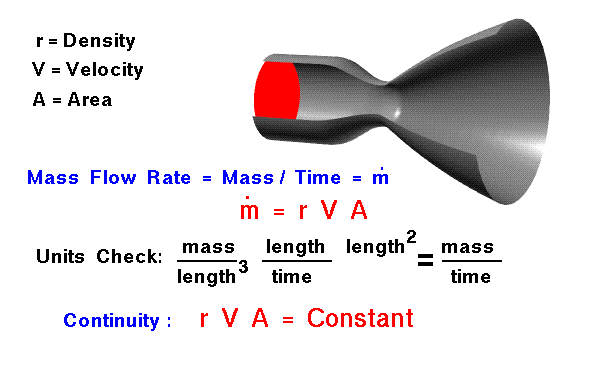+ Text Only Site
+ Non-Flash Version
+ Contact Glenn## Mass Flow Rate

### Glenn Research CenterThe conservation of mass is a fundamental concept of physics. Within some problem domain, the amount of mass remains constant --mass is neither created nor destroyed. The mass of any object is simply the volume that the object occupies times the density of the object. For a fluid (a liquid or a gas) the density, volume, and shape of the object can all change within the domain with time. And mass can move through the domain. On the figure, we show a flow of gas through a constricted tube. There is no accumulation or destruction of mass through the tube; the same amount of mass leaves the tube as enters the tube. At any plane perpendicular to the center line of the tube, the same amount of mass passes through. We call the amount of mass passing through a plane the mass flow rate. The conservation of mass (continuity) tells us that the mass flow rate through a tube is a constant. We can determine the value of the mass flow rate from the flow conditions.

If the fluid initially passes through an area A at velocity V, we can define a volume of mass to be swept out in some amount of time t. The volume v is:

v = A * V * t

A units check gives area x length/time x time = area x length = volume. The mass m contained in this volume is simply density r times the volume.

m = r * A * V * t

To determine the mass flow rate mdot, we divide the mass by the time. The resulting definition of mass flow rate is shown on the slide in red.

mdot = r * A * V

How do engineers use this knowledge of the mass flow rate? From Newton's Second Law of Motion, the aerodynamic forces on an aircraft (lift and drag) are directly related to the change in momentum of a gas with time. The momentum is defined to be the mass times the velocity, so we would expect the aerodynamic forces to depend on the mass flow rate past an object. The thrust produced by a propulsion system also depends on the change of momentum of a working gas. The thrust depends directly on the mass flow rate through the propulsion system. For flow in a tube, the mass flow rate is a constant. For a constant density flow, if we can determine (or set) the velocity at some known area, the equation tells us the value of velocity for any other area. If we desire a certain velocity, we know the area we have to provide to obtain that velocity. This information is used in the design of wind tunnels.

Considering the mass flow rate equation, it would appear that for a given area, we could make the mass flow rate as large as we want by setting the velocity very high. However, in real fluids, compressibility effects limit the speed at which a flow can be forced through a given area. If there is a slight constriction in the tube, as shown in the nozzle graphics, the Mach number of the flow through the constriction cannot be greater than one. This is commonly referred to as flow choking and the details of the physics are given on a page considering compressible mass flow rates.

Activities:Guided Tours

Navigation ..Beginner's Guide Home Page+ Inspector General Hotline + Equal Employment Opportunity Data Posted Pursuant to the No Fear Act + Budgets, Strategic Plans and Accountability Reports + Freedom of Information Act + The President's Management Agenda + NASA Privacy Statement, Disclaimer, and Accessibility CertificationEditor: Nancy Hall NASA Official: Nancy Hall Last Updated: May 13 2021 + Contact Glenn